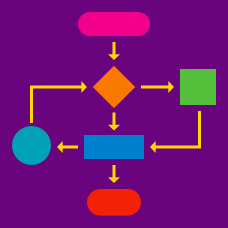Computer Science

# Data Structures Warmup

Starting from the root node of the tree (marked in purple) what is the maximum possible number of steps needed to reach another node in the tree?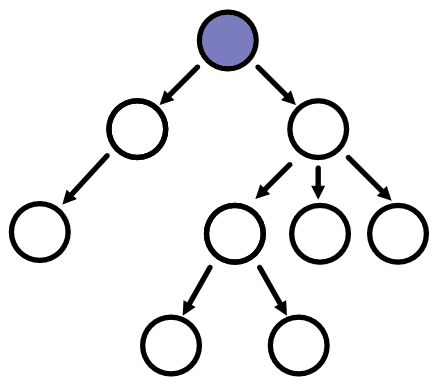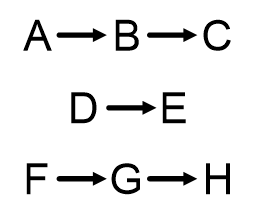Three linked lists are shown above.

If two new pointers $$H\rightarrow A$$ and $$E\rightarrow F$$ are added a single linked list is formed. What does it look like?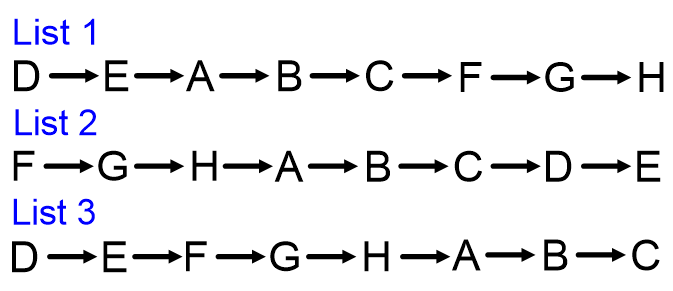In a binary search tree, when an entry is added, the algorithm starts at the root node, and then moves right if the entry is larger than the current node or left if the entry is smaller than the current node. If there is no node available in the specified direction, the entry is added in that position.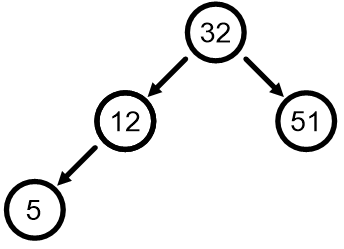If the number 40 were added to the tree above, what would the new tree look like?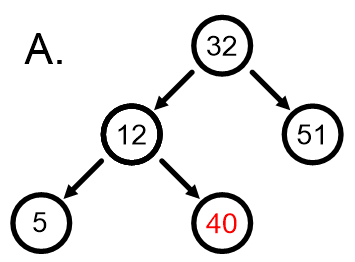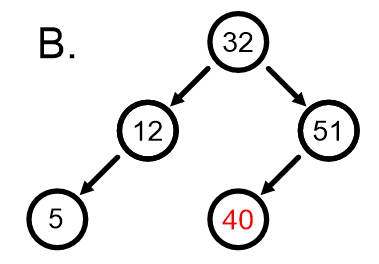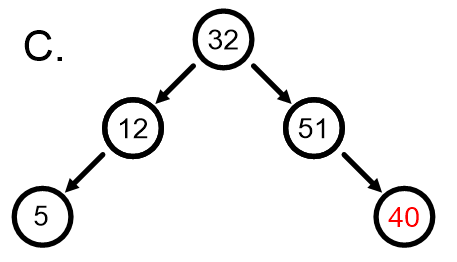An abstraction of a two-dimensional array structure is shown below; a black square indicates a 1 and a white square indicates a 0.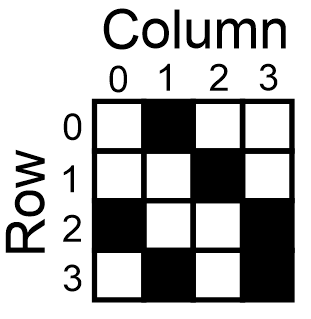A loop runs through all entries on row 2 and sets each $$A[\text{row}][\text{column}]$$ to equal $$A[\text{row} + 1][\text{column}]$$. What will the new array look like?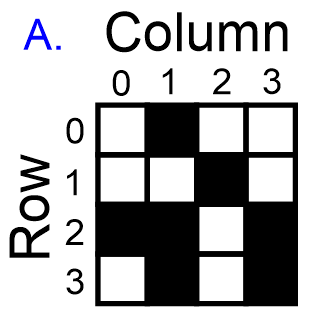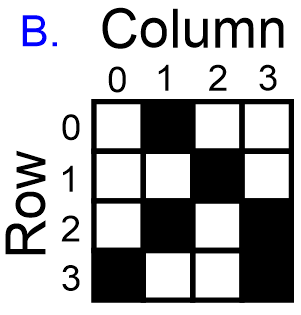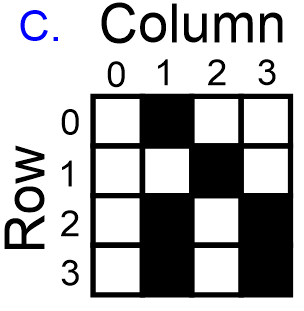Data entered into a stack data structure is stored much like a physical stack; the first entry in is the last entry out.

Suppose you have a stack where you place (or "push") the numbers 5, 9, 13, and 15 in that order.

If you then extracted (or "popped") two numbers, what would the second number that you popped be?

×